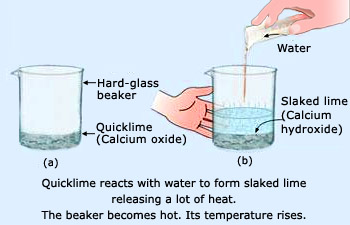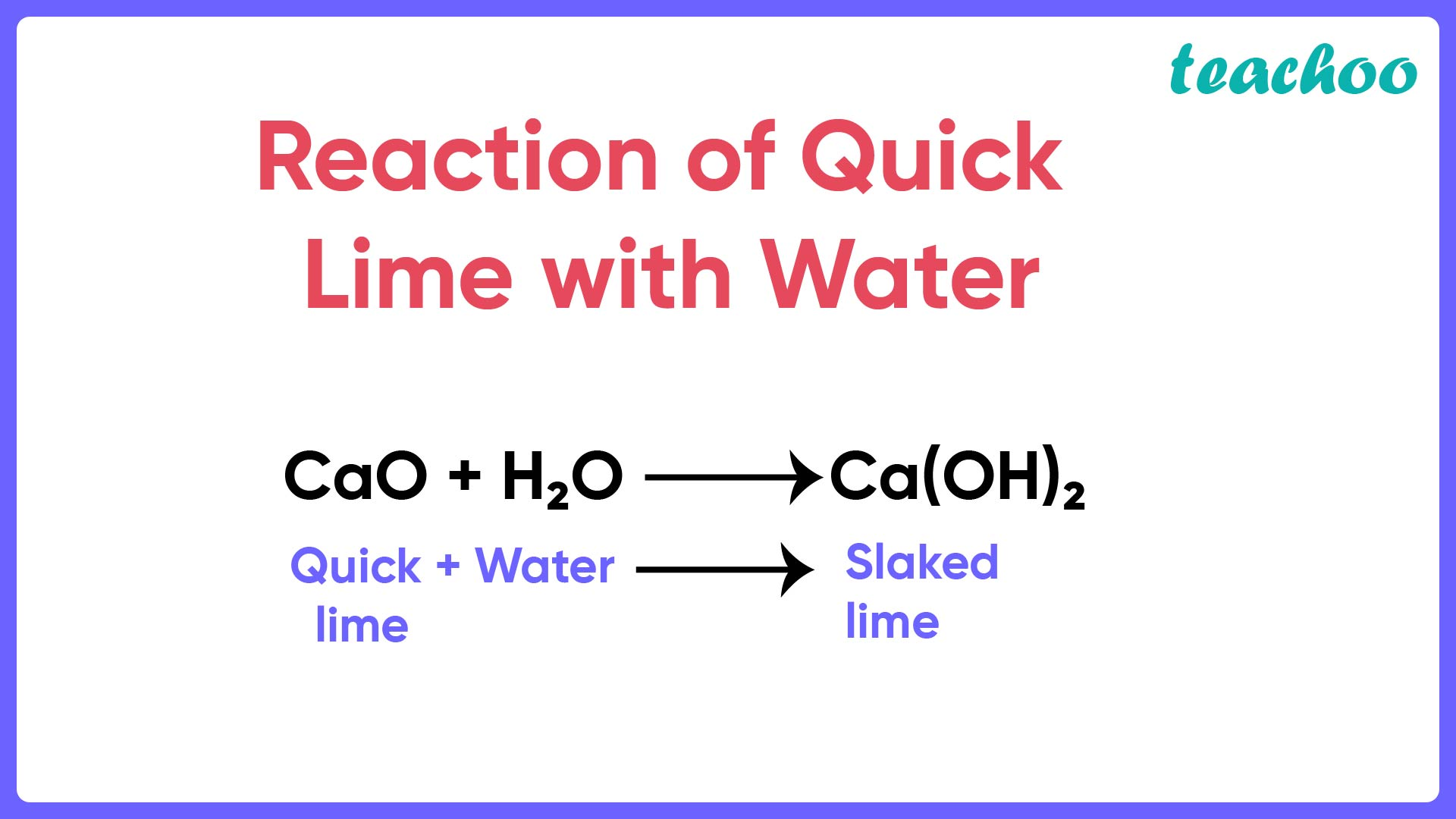Case Based Questions (MCQ)

Class 10
Chapter 2 Class 10 - Acids, Bases and Salts

## Suhana wanted her house to be white washed. She  bought 10 kg of quicklime from the market and  dissolved it in 30 L of water. On adding lime to  water, she observed that the water started boiling  even when it was not being heated.## (iv) Calcium carbonate

Ans.

When water is added to quick lime, slaked lime i.e. Calcium hydroxide is formed.

The reaction is as follows:So, the correct answer is (ii) Calcium hydroxide

## (iv) Calcium carbonate

Ans.

The common name for quick lime is Calcium oxide

So, the correct answer is (ii)

## (iv) Ca 2 OH 2

Ans.

The correct formula for calcium hydroxide is Ca(OH) 2

So, the correct answer is (iii)

## (iv) The pH of the resulting solution will be less  than seven.

1. I and II
2. II and III
3. I and IV
4. III and IV

Ans.

Here,

• Exo means out and thermic means heat. Exothermic reactions are the ones in which heat is released .
• Endo means in and thermic means heat. Endothermic reactions are the ones in which heat is absorbed .

Since

• Heat is given out when quick lime reacts with water and,
• Ca(OH) 2 is formed which is basic in nature,
• The reaction is exothermic and pH of resulting solution will be more than 7

So, the correct option is (II)

Learn in your speed, with individual attention - Teachoo Maths 1-on-1 Class